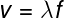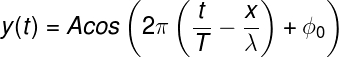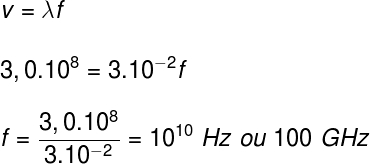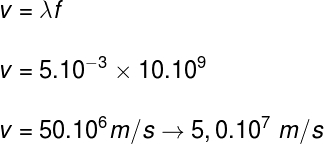Electricity & Megnetism

# Electromagnetic waves

Electromagnetic waves transport energy through space without transporting matter. This type of wave is formed by electric and magnetic fields perpendicular to each other, in addition, it does not need a medium material to propagate. In a vacuum, electromagnetic waves propagate at the speed of light , about 3.0 x 10 8 m/s.

## What are electromagnetic waves?

An electromagnetic wave is formed by oscillating electric and magnetic fields perpendicular to each other. . The propagation direction of the electromagnetic wave, in turn, is perpendicular (90º angle) to the electric and magnetic field vectors.

Due to the angle formed between the disturbance and the propagation direction, electromagnetic waves are characterized as transverse . Like all waves, they can transfer energy between different points in space, but they do so without any transport of matter, as they are not capable of doing so.

Electromagnetic waves have the following characteristics: wavelength propagation speed amplitude and frequency, and are liable to undergo any type of wave phenomenon known, such as reflection , refraction , polarization , diffraction , scattering, absorption and interference .

Electromagnetic waves, formerly known as “Hertzian” waves, were discovered by the German physicist Heinrich Rudolf Hertz , who, in 1883, knew how to produce them. The first complete and satisfactory explanations for the nature of these waves equations came shortly afterwards, after the work of James Clerk Maxwell , who unified electrical and magnetic phenomena into wave equations now known as Maxwell ‘s .

## Characteristics of electromagnetic waves

Understand the characteristics of electromagnetic waves:

• Amplitude: concerns its intensity , that is, the amount of energy that these waves are able to transfer.
• Velocity: depends exclusively on the medium in which they are found. For example, in water, the propagation speed of electromagnetic waves is slower than in air, due to the phenomenon known as refraction .
• Frequency: is the measure of complete oscillations that these waves perform every second. In the SI , the unit of measurement for frequency is the hertz (Hz). Furthermore, it is known that the frequency is determined by the inverse of its period, namely: the time required for an electromagnetic wave to complete an oscillation.
• Wavelength: it is the space through which the wave propagates until a complete oscillation is formed, it is also the distance between two positions of maximum amplitude (crests) and of minimum amplitude (valleys).

If you want to delve deeper and get more information about the general characteristics of waves, whether mechanical or electromagnetic, we suggest you check out our article on waves .

## Types of electromagnetic waves

Electromagnetic waves are classified by their frequency or based on their wavelength . Although there is no clear distinction between one type of electromagnetic wave and another, there is a continuum of frequencies known as the electromagnetic spectrum .

The electromagnetic spectrum concerns the entire range of possible frequencies that an electromagnetic wave can present, the distinction between them, however, is phenomenological, for example: although they are electromagnetic waves, x-ray and visible light are quite different. in the way they interact with matter. Check out the types of electromagnetic waves and discover how they are present in our daily lives:

• Radio waves : have the lowest frequency among electromagnetic waves and, consequently, the longest wavelength. They are commonly used for television, radio and cellular signal transmission.
• Microwaves : they have a slightly higher frequency than radio waves, they are widely used in telecommunications (on wi-fi, for example) and also in radars that capture the speed of moving vehicles.
• Infrared : has afrequency slightly lower than that of visible light, this type of wave, also known as a heat wave , is capable of increasing the thermal agitation of atoms and molecules. When we approach a fire and feel its heat, part of the energy transmitted to us comes in the form of thermal radiation , carried by infrared waves.
• Visible light: is the one that can sensitize the eyes of human beings, since there are animals capable of seeing different types of electromagnetic waves, such as infrared and ultraviolet, for example. Visible light comprises a narrow range of wavelengths in the electromagnetic spectrum, between 700 nm and 400 nm (nanometers = 10 -9 m).
• Ultraviolet : it is considered an ionizing radiation, that is, during its interaction with matter, it is capable of ripping electrons from atoms, causing damage to important molecules, such as those present in the DNA of epithelial cells . Due to its ionizing capacity, ultraviolet radiation is used in the sterilization of medical devices, for example.
• X-rays: are ionizing electromagnetic waves with great penetrating power. This type of wave is able to cross different types of tissues, thanks to its short wavelength. They are widely used in imaging tests, such as radiography and tomography.
• Gamma rays: are the highest frequency electromagnetic waves in the entire electromagnetic spectrum, they can be obtained in nuclear reactions and during pair annihilation (when there is contact between matter and antimatter). However, most of the gamma rays that fall on Earth come from stars , such as our Sun. This type of radiation is extremely penetrating and has great ionizing capacity.

## Electromagnetic wave formulas

When we refer to electromagnetic waves, there are some formulas relevant to your study, check them out.

## Wave speed, frequency and wavelength

This formula relates the propagation speed of the electromagnetic wave to its frequency and wavelength:v – propagation speed (m/s)

λ – wavelength (m)

f – frequency (Hz)

Since the common frequencies of electromagnetic waves are usually very large numbers, it is common to use prefixes to refer to these orders of magnitude . Check out which are the most used unit prefixes to represent the frequency of these waves:

 Frequency order of magnitude and symbol prefixed frequency name 10 3 Hz – 1 kHz 1 kilohertz 10 6 Hz – 1 MHz 1 megahertz 10 9 Hz – 1 GHz 1 Gigahertz 10 12 Hz – 1 THz 1 terahertz

Prefixes used for electromagnetic wave frequencies

Since the wavelength is inversely proportional to the frequency of the electromagnetic wave , it is common to use some prefixes to denote wavelengths whose orders of magnitude are very small. Check out some of the most used:

 Wavelength order of magnitude and symbol prefixed frequency name 10 -3 m – 1 mm 1 millimeter 10 -6 m – 1 μm 1 micrometer 10 -9 m – 1 nm 1 nanometer 10 -12 m – 1 pm 1 picometer

## harmonic wave equation

In the case where an electromagnetic wave can reflect in a cavity and produce a constructive interference on itself , a harmonic, or stationary wave, will be produced. The equation used to describe the oscillation of this type of wave is shown below, check it out:y(t) – vertical position of the wave as a function of time (m)

THE – maximum wave amplitude (m)

t – instant of time(s)

T – period of the wave (s)

x – horizontal position of the wave (m)

λ – wavelength (m)

φ 0 – initial phase of the wave (rad)

## Exercises on electromagnetic waves

Question 1) An electromagnetic wave of wavelength 3.0 cm travels in vacuum with a speed of 3.0 x 10 10 8 m/s. The frequency of this electromagnetic wave is equal to:

a) 100 GHz

b) 30 MHz

c) 200 THZ

d) 50 GHz

e) 600 GHz

Template: letter a

Resolution:

To calculate the frequency of this electromagnetic wave, we must use the formula that relates speed, wavelength and frequency, check:According to the calculations made, the frequency of this wave is 1010 Hz, which can also be written as 100.10 9 Hz or 100 Ghz.

question 2)An electromagnetic wave propagates through a medium material with wavelength and frequency equal to 5 mm (0.005 m) and 10 GHz, respectively. Determine the speed of propagation of this wave.

a) 1.5.10.10 8  m/s

b) 5.0.10 9 m/s

c) 3.0.10 8 m/s

d) 5.0.10 7 m/s

e) 6.0.10 4 m/s

Template: letter d

Resolution:

Using the wave velocity formula, it is possible to obtain the following result, check: